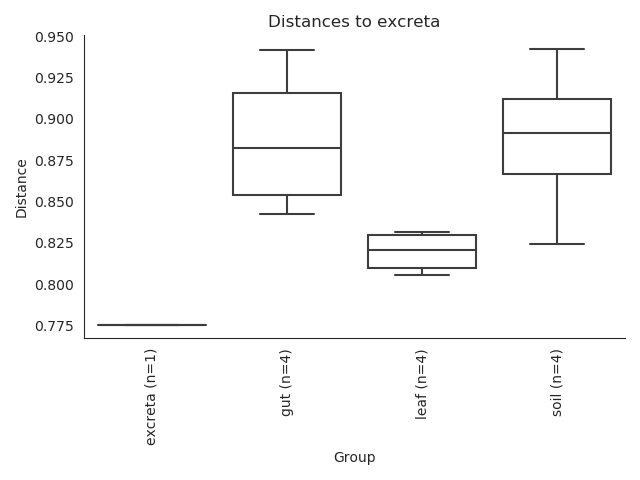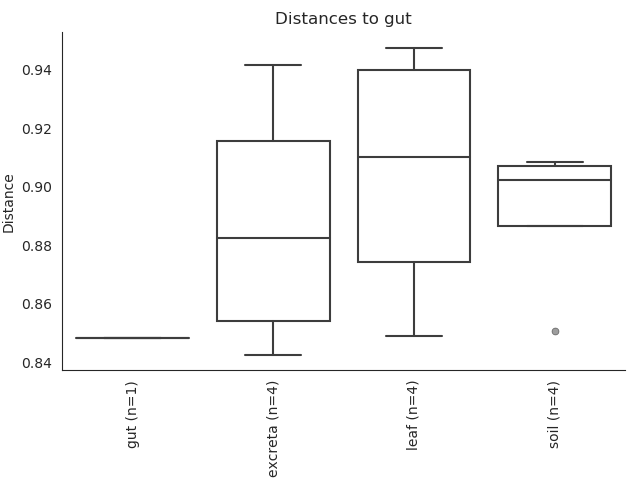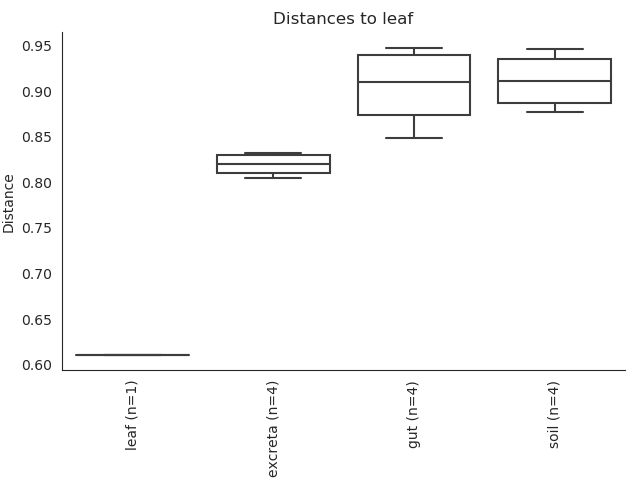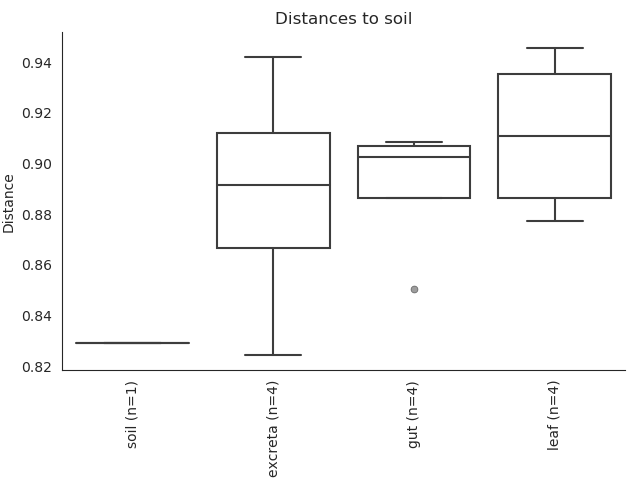# interpretation of beta-diversity-group-significance box plots

Hello all,
Can anyone pls help me to interpret my beta-diversity-group-significance results?
I have 4 groups each containing 2 samples. total number of sample is 8. I am unable to understand why in all the four plots, the first box has appeared to be a line only. Did I make any mistake?Any kind of help is highly appreciated.
Best regards,

Hi @Archana,

Welcome to theforum!

There aren't any glaring beta-diversity related mistakes, but, let's step back and start talking about beta diversity. So, when we measure beta diversity, we measure a distance. A distance always has to be between two points. (The distance from->; the distance from->; the distance from->as a few examples.) For this discussion it doesn't really matter how you measured your distance (although check out the post at the bottom for more about the metrics.)

When you make your beta diversity boxplots, you're plotting the distribution of distances, and the n is the number of distances in the boxplot. So, then, if you have_{1} and_{2}, and_{1} and_{2}, you get the following distances:

• d(_{1},_{2})
• d(_{1},_{1})
• d(_{1},_{2})
• d(_{2},_{1})
• d(_{2},_{2})
• d(_{1},_{2})

If we group the distances, we have one distance that's d(,), one distance that d(,), and four distances that are d(,).... which are exactly the numbers in your boxplot.

The place I might argue you have an issue is therefore not in the math, but in the sample size. A permutative test or kruskal-wallis won't work well with these sample numbers. They're just too small for statistics.

Best,
Justine

7 Likes

Hi Justine,
The way you’ve explained it is just wow! Thanks a lot.4 Likes

This topic was automatically closed 31 days after the last reply. New replies are no longer allowed.